# High School Math : Pyramids

## Example Questions

2 Next →

### Example Question #5 : How To Find The Surface Area Of A Pyramid

What is the surface area of a square pyramid with a height of 12 in and a base side length of 10 in?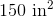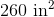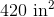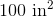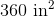Explanation:

The surface area of a square pyramid can be broken into the area of the square base and the areas of the four triangluar sides. The area of a square is given by: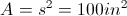The area of a triangle is: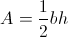The given height of 12 in is from the vertex to the center of the base. We need to calculate the slant height of the triangular face by using the Pythagorean Theorem: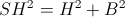where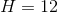and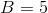(half the base side) resulting in a slant height of 13 in.

So, the area of the triangle is: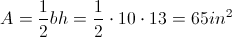There are four triangular sides totaling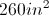for the sides.

The total surface area is thus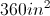, including all four sides and the base.

2 Next →